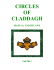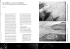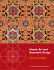# japanese Temple Geometry Problems

## Transcription

japanese Temple Geometry Problems
```I
n the opening paragraph of their book japanese Temple Geometry Problems , H. Fukagawa and
D. Pedoe write "During the greater part of the Edo period (1603-1867) japan was almost completely
cut off from the western world.
Books on mathematics, if they entered japan at all, must have been
scarce, and yet, during this long period of isolation people of all social classes, from farmers to samurai,
produced theorems in Euclidean geometry which are remarkably different from those produced in the
West during the centuries of schism, and sometimes predated these theorems by many years."
As far as I know,  is the only extensive publication in English on the subject of japanese geometry,
and this book and the many books on the subject in japanese consist mainly of collections of problems,
•
•
••
•
•
•
••
•
••
•
••
•
•
•
••
•
•
•
••
•
•
•
••
•
•
•
•
••
••
••
•
••
•
•
••
•
••
•
•
•
•
•
•
•••
•
with or without solutions. Therefore there are many questions to which I still do not know the answers.
How much Western mathematics came to japan before 1600, from where, and in what form? Did the
Japanese have books of mathematical theory and techniques? The authors of  say that there were few
colleges or universities in Japan during the period in question, but many private schools.
The problems, mainly geometrical, were either published in books or painted on wooden tablets containing
text and coloured figures and hung in shrines and temples; hence the use of the term "temple geometry"
(a term not used in japan) although twice as many tablets appear in shrines as in temples. The word
for Japanese mathematics is wasan, and the tablets are called sangaku. Collections of sangaku problems,
with solutions, appeared in japanese books in the 18th and 19th centuries, and there are modern books
of collections of old problems .
It is my impression that this japanese geometry differs from Western geometry mainly in the type of
problem that seems to have interested and intrigued the Japanese geometers. The japanese love of
artistic design is evident in many of the figures.
Much of my information about the subject has been gained from Hiroshi Okumura, an associate
professor at the Maebashi College of Technology, in Gunma Prefecture. We correspond regularly and
have discussions at conferences. A few years ago he gave me a copy of The Sangaku in Cunma ,
published in 1987 by the Gunma Wasan Study Association of which he is a member. This book is a
limited edition, beautifully printed, bound and illustrated. It is sometimes possible to understand a
problem from its illustration without being able to read the japanese text.
The text of wasan was usually written in Kanbun, which is based on Chinese (since the japanese
language was written mainly in Chinese characters, I presume this means that Kanbun uses Chinese,
rather than japanese, grammar and syntax). Kanbun cannot be read by most people in modern japan.
Many of the books on wasan, including [1 L are like sets of "M iscellaneous Exercises" at the end of a
geometry textbook, but with the rest of the textbook missing. I propose here to give a few examples of
such exercises of varying degrees of difficulty, to provide a flavour of the types of problem encountered
in wasan. I am not giving solutions here, but my comments may be helpful. Some of the problems have
been used in secondary school mathematics clubs, or have provided problems or articles in pedagogical
•
•
••
•
•••
•
•
•
••
•
••
••
••
•
••
•
••
••
•
••
•
••
••
•
••
•
•
•
••
••
••
•
journals .
1. Given the radius of the large semicircle in figure 1, find the radii of the
other circles and semicircles [2, p.67] .
If the radius of the large circle is 6, the other radii are 3, 2 and 1. Once
the solution has been obtained, we observe that the centres of the circles
and semicircles form various 3-4-5 triangles, and the figure can be built
up by drawing the small circles first, then circumscribing the large circle .
Figure 1
ID
EOLEY
'
Mathematical
2. In figure 2, which is bounded by a square, show that the two upper
circles have the same radius as the three lower circles.
This problem and the next were found by Hiroshi Okumura in a book of
wasan problems in the Library of Congress, Washington . If the square
has side 6, once again we have a 3-4-5 triangle, and we have to prove that
the radius of its inscribed circle is 1. This fact is not well known. My
original proof used areas: the triangle IBC is figure 3 has base BC and height
r so its area is BC.r/2. Similarly the areas of ICA and lAB are CA.r/2 and
AB.r/2. But the area of the right angled triangle ABC is BC.CA/2; hence
BC.CA = (BC + CA + AB)r.
Figure 2
Putting BC
•
•
•
from C have length r. Putting y + r
BC
we deduce that r
= 1.
= 3,
r+x
= 4,
x +y
=5
we deduce that r
= 1.
= y + r,
CA
=r
+ x, AB
= x + y in
8
(1) we obtain
r 2 + rx+ ry+ xy= (2r+ 2x+ 2y)r;
if we multiply by 2, this can then be rewritten as
(r + x)l + (r + y)l
•
••
•
•
••
••
=5
Suppose now that figure 3 shows a general right angled triangle. Putting
•
••
•
••
•
••
•
••
•
•
•
AB
x say, and the tangents from B have equal length y say; because of the right angle at C the tangents
••
•
•
•
•
••
•
••
•
•
= 4,
CA
A colleague of mine suggested a simpler proof. The tangents from A to the incircle have equal length
•
••
•
••
••
•
•
••
= 3,
(1)
= (x
+ y) 2,
and so we have a proof of Pythagoras' theorem using the incircle, which
may not be new but I have not seen it before .
Finally on this topic, suppose the sides of a right angled triangle have
integer lengths a
= y + r,
b
so it is easy to prove either
Hence r
= (a
= r + x,
a, b, c
c
=x
Figure 3
+ y; then a 2 + b 2 = c 2
are all even or only one of them is even .
+ b - c)/2 is an integer.
r--:::o>-~----;;;--......_;:--;5
3. In figure 4, all three circles inside the rectangle have equal radius .
Show that the lengths RQ and RT are equal.
We can also ask: what is the ratio of the sides of the rectangle? The
figure has been drawn inaccurately so as not to reveal the answer.
This problem can be generalised, as stated below, to the case where
the interior line does not pass through the vertex R.
R
Q
Figure 4
4. In figure 5, all three circles inside the rectangle have equal radius .
Show that the lengths RQ and UT are equal.
For a solution of problems 3 and 4, see  .
•
•
••
••
•
U
Q
Figure 5
r.r:11 Mathematical
W..
ED~EY
SEPTEMBER 1997
R
••
•
••
••
•
•
••
•
••
••
•
••
••
••
•
••
•
•
••
••
•
••
•
•
•
•
••
•
5. In figure 6, two circles of equal radius a touch the outer circle (of radius r)
at opposite ends of a diameter, and the same is true of the circles of radii
b and c. Show that a + b + c = r.
This problem, with a long solution, appears in a collection of wasan problems
. Hiroshi Okumura has found a simple solution by observing that the
centres of the seven circles form the vertices of six congruent triangles with
sides of lengths b + c, c +
a, a + b.
As in problem 1, the smaller circles
can be drawn first, with a final circle of radius r circumscribing them  .
Many wasan problems involve touching circles, as a glance at the figures
in  and  will show, but many do not. Before giving examples of other
types of problem, it is worth mentioning two well known results. A problem
Figure 6
on a tablet from 1796, since lost, asked for a formula connecting the radii
of four circles each of which touches the other three [1, p. 90]; such a formula was given earlier
by Descartes. The corresponding problem for five spheres, each of which touches the other four,
appeared on a tablet in 1785, and a solution was given in a woodblock-printed book in 1841. This
solution required six pages: the powerful technique of inversion was apparently
not known in japan at the time [1, p.160, pp.179-186] .
6. In figure 7, which is based on five squares, show that the area T of the triangle
and the area S of the square are equal [1, p.48] .
There must be tedious ways of proving this using excessive tri gonometry or
coordinate geometry, but it is a challenge to find a solution that is visually clear.
The problem, with my solution, appears in  .
Figure 7
7. In figure 8, find the centre of gravity of the solid semi-ellipsoid of
revolution, formed from two ellipses with common major and
minor axes.
The figure comes from [2, p.157], and is redrawn by kind
permission; my original guess, that it shows half a cocon ut hung
up for birds to feed on (a common practice in the U.K.), was not
far short of the mark!
Figure 8
8. In figure 9 a rectangle of paper is folded so that
opposite corners coincide. If the longer side of
the rectangle is of given fixed length, what shape
of rectangle gives the greatest value of the area
of ABC?
8
This problem comes from [2, p.188]. The original
figure merely shows two folded sheets of paper,
red on the inside and white on the outside, each
inserted in the top of a wooden stick which is
placed in a small jar of rice wine; the two jars
rest on a table. The problem cannot be deduced from this figure
without a translation of the text!
Figure 9
•
•
•
•
•
••
•
•
••
•
•
••
•
••
•
••
•
••
•
•••
•
••
•
••
•
•
••
athematical
MEOl.EY
.n
~
••
•
••
•
••
•
•
•
•
••
•
••
•
•
The solution of the previous problem requires elementa ry ca lcu lu s. A remark in [1, p.138] mentions
the only known reference to a parabola, from 1844, in which ca lculus is involved, so the methods
of ca lcu lu s must have been ava il able. There is also on ly one reference to a hyperbola; this is
apparently because the japanese regarded an ellipse as a section of a right circular cyl inder rath er
than of a cone, and a hyperbola cannot be obta ined in this way. But problems involving ellipses
abound. The next examp le is so simple and elega nt that I am surprised that I have not found it in
any western text on conic sections.
9. let POR and QOS be perpendicular diameters
of an ellipse, as in figure 10. Then PSRQ is a
rhombus, which has an inscribed circle with
centre 0. Show that the radius of this circle is
independent of the two diameters (1841 ); [1, p.68] .
•
••
•
•
••
•
••
•
•
••
•
•
•
••
•
••
•
••
•
••
••
•
••
••
••
•
••
•
•
•
•
••
•
••
••
•
••
•
The solution given in [1, p.159], using coord inate
geometry, can be shortened. We make use of a
lemma that is also of interest in its own right.
lemma
In figure 11 suppose the axes of the ellipse have lengths
2r and 25. Then
r 1 + 5-' = a-' + b ' .
Proof
If we use the axes of the ellipse as coordinate axes, its equation is
The points R and S with coo rdin ates
8, r si n 8 ) and
(5 sin
lie on the ellipse; hence
so that 1/ r ' =
cos 1 8 /a' +
-5 cos
r'cos' 8 /a ' +
sin 28
5 _, =
8)
r 2sin 2 8
/b'
=
/b' ;
Figure 11
a 2 + b-2 .
Now in figure 12 let the radius of the inscribed circ le be d, and
ways as r5/2 or td/2. Hence r252 = t 2 d 2 = (r' + 52 )d 2 • Hence
d -' = r-' +
5
2
=
a 2 + b-2
by the lemma. So the radius
d is
independent of the diameters POR and QOS.
0
Note that the corresponding resu lts d-' =
true for the hyperbola
Fi gure 12
SEPTEMBER 1997
r2+
8,
write RS = t. The area of triangle ROS can be calculated in two
•
••
•
••
•
n11 Mathematical
~ EDLEY
2a and 2b,
and suppose the perpendicular diameters POR and QOS have lengths
cos
••
••
••
•
••
•
••
••
••
Fi gure 10
(r
•
•
•
•
•
••
•
••
•
••
•
•
••
x'/a
2
-
y'/b '
r2+ 5
1
=
a-' - b -2 remain
= 1 as long as b 2 >
a' .
••
•
••
The next two problems were brought to my attention by Hiroshi Okumura. Problem 11 which dates
from 1845 [2, p.72] is a generalisa tion of ProblefT11 0 which dates from 1878 [1, p.5], so Problem 10
probably appeared somewhere else at an earlier date.
10. If r denotes the radius of the small circle in figure 13, show that
1/r
= 1/ff +
A
1/AF.
11. If r and s denote the radii of the two small circles in figure 14,
show that
1/..frS
= 1/ff +
1/AF.
It is not difficult to prove 10 by coordinate
A
geometry, but the calcu lat ions involved in
investigating 11 are more difficult, and I eventually
began to suspect that it is not true. In fact it is
possible to show, by cons idering specia l cases of
Figure 13
figure 14, that the express ion {;; (1/FE + 1/A F)
ca n take al l positive va lues . Why then should such an incorrect conjecture
have been made? We have to make al lowances for inaccurate measurements
even in a good drawing, and it appears that F has to be very close to the
bounding circle before the va lue of{;; (1/Ff + 1/AF) differs appreciably from 1.
M
2
Figure 14
References
1. H. Fukagawa and D. Pedoe, japanese Temple Geometry Problems, The Charles Babbage Research Centre,
2. Gunma Wasan Study Association, The Sangaku in Cunma (in Japanese), 1987.
3. Okayu (ed. ), revised Ogawa, Sampo Semmon Sho, 1940.
4. H. Okumura, Circle patterns arising from a seven-circle probl em, Crux Mathematicorum 21 (1995) 213-217.
5. J. F. Rigby, Cirlce problems arising from Wasan, Symmetry: Culture and Science (to appear).
6.
J. F. Rigby, An incorrect sangaku conjecture, Symmetry: Culture and Science (to appear).
7. ]. F. Rigby, A sangaku prob lem, Mathematics and Informatics Quarterly (to appear).
8. Sakuma, Sampo Kigen Shu Vol 2, 1877.
He recently retired from his position of Reader in Pure Mathematics at the
University of Wales, Card iff, but is still lecturing on geometry part-time. He has
been based in Card iff since 1959, and has held temporary university appointments
in Toronto, Ankara and Singapore. His hobbies include ornamental art, classical
music, folk dancing and mountain wa lking.
Dr. Rigby ca n be co ntacted at
University of Wales, School of Mathematics,
Senghennydd Road, P. 0. Box No. 926, Cardiff CF2 4YH, UK.
e-mail: [email protected] uk
athematical .-r!l
ME0LEY
.0.0:..
```

### Japanese Family Crests (Mon)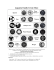### The New Temple Geometry Problems in Hirotaka`s Ebisui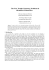### Japanese Temple Geometry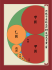### Crop Circles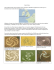### Kamaitachi _ Hosoe Eikoh, Keene Donald, Hijikata Tatsumi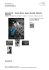### 食道楽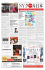### PDF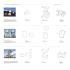### ILA Conference Center, GOLF Thursday, 2 June 2016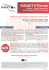### Japanese Water Iris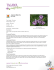### 20160121 smile通信vol.06英語版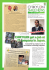### In Vivo - Sinerga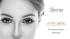### Asian Puppet Theatre Exchange - Japan Foundation Kuala Lumpur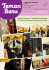### Foodmaster Plaza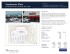### PDF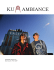### 2016 Annual Conference Program Schedule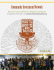### Geometric Ornament in Art and Architecture of Western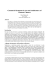### Chem 111 Summer 2011 Key II Whelan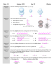### another dutch eyewitness sees a crop circle forming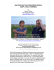### Sacred Mathematics: Japanese Temple Geometry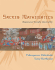### circles of claddagh - St. Patrick Catholic Church